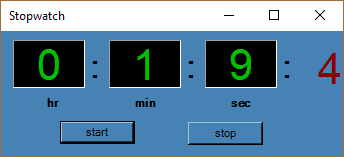Stopwatch Application in Visual Basic.NetPosted by Samath1810 December 28, 2016

A stopwatch program measure the amount of time elapsed from a particular time when it is activated to the time when it is deactivated.Code:

```Public Class Form1
Inherits System.Windows.Forms.Form
Dim sec, min, msec, hr As Integer

Private Sub Button1_Click(ByVal sender As System.Object, ByVal e As System.EventArgs) Handles Button1.Click
Timer1.Start()
Timer1.Interval = 100
msec = 0
sec = 0
min = 0
End Sub

Private Sub Button2_Click(ByVal sender As System.Object, ByVal e As System.EventArgs) Handles Button2.Click
Timer1.Stop()
End Sub

Private Sub Timer1_Tick(ByVal sender As System.Object, ByVal e As System.EventArgs) Handles Timer1.Tick

If msec = 10 Then
sec = sec + 1
msec = 0
End If

If sec = 60 Then

min = min + 1
sec = 0
End If
If min = 60 Then
hr = hr + 1
min = 0
End If

Label6.Text = hr
Label3.Text = min
Label2.Text = sec
Label1.Text = msec

msec = msec + 1
End Sub

End Class```